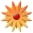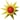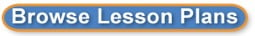# Math Technology Lesson

Page Views: 2931

Email This Lesson Plan to Me
Overall Rating:(5.0 stars, 1 ratings)

 Subject(s): Grades 7 through 8 School: Grantham Acad for Engineering, Houston, TX Planned By: Shelli Nunciato Original Author: Shelli Nunciato, Houston
Subject: Math Grade Level: 7th Time Frame: Through year to introduce and review concepts

TEKS:
(1) (B) convert between fractions, decimals, whole numbers, and percents mentally, on
paper, or with a calculator
(2) (A) represent multiplication and division situations involving fractions and decimals
with models, including concrete objects, pictures, words, and numbers;
(3) (A) estimate and find solutions to application problems involving percent; and
(B) estimate and find solutions to application problems involving proportional relationships such as similarity, scaling, unit costs, and related measurement units.
(6) (A) use angle measurements to classify pairs of angles as complementary or
supplementary; (B) use properties to classify triangles and quadrilaterals; (D) use critical attributes to define similarity.
(9) (A) estimate measurements and solve application problems involving length
(including perimeter and circumference) and area of polygons and other shapes;
(13) (A) identify and apply mathematics to everyday experiences, to activities in and
outside of school, with other disciplines, and with other mathematical topics.

Overview/Purpose:
Using technology and gathered photos, calculate fractions, decimals, and percents; analyze angles, triangles, and quadrilaterals; and find length and area of objects in and out of school.

Objectives:
1. convert fractions to decimals, whole numbers, and percents from photos
2. multiply and divide fractions and decimals from photos
3. estimate and calculate percent, scaling, and unit costs from photos
4. categorize angles, triangles, and quadrilaterals from photos
5. estimate and calculate length and area of shapes from photos

Lesson Methodology: Using images collected by instructor, teacher models (eliciting responses from class)
1) converting fractions to decimals, whole numbers, and percents from photo of lockers
2) multiplying and dividing fractions and decimals from photo of lockers
3) estimating and calculating percent, scaling, and unit costs from photo of textbook closet
4) categorizing angles, triangles, and quadrilaterals from photos of ceiling and flooring tiles
5) estimating and calculating length and area of shapes from photos of different types of light fixtures

Guided Practice: Using the same photos from the lessons, students will work in groups to calculate similar problems but with different numbers.

Individual Practice: In partners, students will gather their own photos and create their own problems with solutions. Partners will then trade photos and questions with another pair of students to answer the student-created questions. Partnerships will swap back to grade. The two partnerships will discuss any discrepancies.

Materials Needed:
1. photos of objects in and out of school
2. digital cameras (1 per 2 students in class)